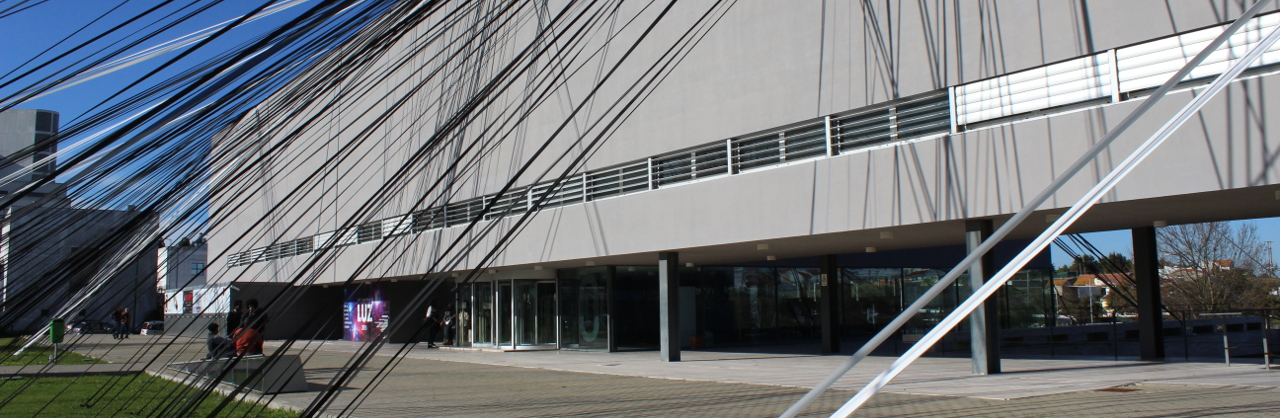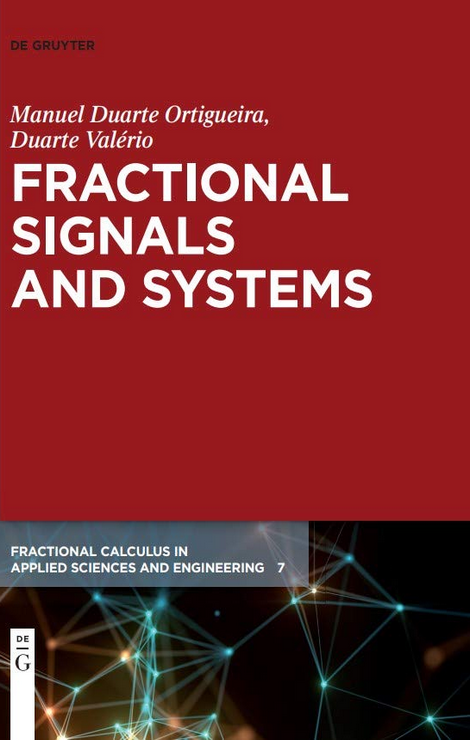# Fractional Signals and Systems##### Autor

Manuel Duarte Ortigueira | Duarte Valério

##### Resumo

This book has a twofold objective: to bring the methodologies of Signal Processing (SP), namely the theory of linear systems, to the Fractional World, and to introduce fractional derivatives and anti-derivatives in the theory and practice of Signals and Systems.

In a simplistic vision, Signal Processing is Mathematical Engineering. It is a like a “marriage” of many mathematical areas with engineering concepts and tools. This brought generality to SP, and allowed it to become the base of many application areas. It currently uses: transforms (Laplace, Fourier, Hankel,...), vectorial, matricial, and tensorial calculus, complex variable functions, set theory, Galois fields, differential and difference equations, graph theory, stochastic processes theory, Statistics, etc.. But, in parallel, it became also a source of new mathematical theories, like Information theory, Wavelet transform and multiresolution. Many numerical tools currently used were developed in SP.

We can trace back the origins of SP to the 17th century, but the most interesting tools were developed by Gauss (he was the first to consider a fast way of computing the Fourier transform) and Fourier (the Fourier series is one of its most importante tools), as well as Prony (who proposed an algorithm for decomposing a function as sum of exponentials, in 1795), Galois (his group theory is the base of the coding in Telecommunications), Euler (he is everywhere; the most interesting transforms are associated to his name), Laplace (probability, transform), Schuster (periodogram, 1898), Heaviside, Carson, Wiener, Kolmogorov, Levinson, van der Pol, and many others.

Meanwhile, and since the forties in the last century, SP evolved and established the bases for the development of many application areas, namely: Telecommunications, Acoustics, Speech, Image, Mechanics, Biomedical Engineering, Economics, Finance, Seismology, Genomics, etc.. Using Mathematics with all the generality and implementing many mathematical tools, it opened the doors of a newworld that came in the last years.

Concerning Fractional Calculus (FC), it dates back to the Leibniz letter exchanges with Bernoulli and others, but its true beginning was with Liouville in 1832 and its developments in the 19th and 20th centuries. During the last 30 years it evolved considerably and became popular in many scientific and technical areas. The progresses in applications vis–à–vis theoretical developments motivated the re-evaluation of past formulations. The concepts of fractional derivative (FD) and fractional integral (FI) assumed various forms not always equivalent and also not compatible with each other. Twenty years ago, the Riemann-Liouville (RL) derivative was currently used; since the last years of past century, the Caputo (C) derivative became preferred, supposedly due to the initial conditions; the Grünwald-Letnikov (GL) derivative was considered a mere approximation to those.

Anyway, there was an intrinsic incompatibility between FC, based on RL and C, and the assumptions currently made in SP. RL and C are unable to support the generalisations of the formalism used in SP. Here, we will try to face the questions involved, and see how to solve the problems.

We separate the text into three parts. The first is dedicated to the study of continuous-time signals and systems, while the second replicates the procedure for the corresponding discrete-time. The third part is dedicated to the presentation of advanced topics.

For both continuous and discrete-time cases,we introduce the concepts of Signals and Systems and present their characterisations. For the case of linear systems, we describe their properties and introduce their input-output relations, stated in terms of the impulse response, transfer function, and frequency response. This is done progressively from the integer order to the fractional order systems. The suitable definitions of fractional derivatives emerge naturally fromthe backward integer/fractional coherence demanded for the systems. Well-known important tools used in daily applications are introduced: feedback, Bode diagrams, stability tests, state-space representations, Laplace transforms, Fourier series and transforms, and Z transform.

In the third part we make a brief study of the system output characteristics when the input is a stochastic process. We include the two-sided fractional derivatives and the fractional Brownian motion. A generalisation of the integer order difference equations is presented to consider fractional delays. Finally, variable order systems are introduced, and a very useful tool for them is presented: the variable order Mittag-Leffler function. The appendices include material on several short topics for reference purposes.

Lisbon, July 2019

Manuel Ortigueira and Duarte Valério

##### ISBN
978-3-11-062129-7